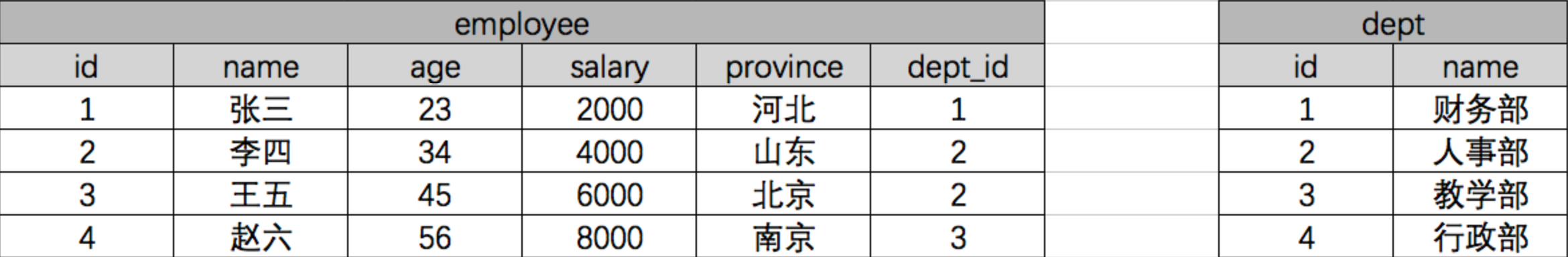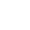/    2019-06-20

# 聚合查询和分组查询

## 聚合

aggregate()是QuerySet 的一个终止子句，意思是说，它返回一个包含一些键值对的字典。

``from django.db.models import Avg, Sum, Max, Min, Count``

``>>> from django.db.models import Avg, Sum, Max, Min, Count>>> models.Book.objects.all().aggregate(Avg("price")){'price__avg': 13.233333}``

``>>> models.Book.objects.aggregate(average_price=Avg('price')){'average_price': 13.233333}``

``>>> models.Book.objects.all().aggregate(Avg("price"), Max("price"), Min("price")){'price__avg': 13.233333, 'price__max': Decimal('19.90'), 'price__min': Decimal('9.90')}``我们使用原生SQL语句，按照部分分组求平均工资：

``select dept,AVG(salary) from employee group by dept;``

ORM查询:

``from django.db.models import AvgEmployee.objects.values("dept").annotate(avg=Avg("salary").values(dept, "avg")``SQL查询：

``select dept.name,AVG(salary) from employee inner join dept on (employee.dept_id=dept.id) group by dept_id;``

ORM查询：

``from django.db.models import Avgmodels.Dept.objects.annotate(avg=Avg("employee__salary")).values("name", "avg")``

``>>> book_list = models.Book.objects.all().annotate(author_num=Count("author"))>>> for obj in book_list:...     print(obj.author_num)...1``

``>>> publisher_list = models.Publisher.objects.annotate(min_price=Min("book__price"))>>> for obj in publisher_list:...     print(obj.min_price)...     9.9019.90``

``>>> models.Book.objects.values("publisher__name").annotate(min_price=Min("price"))<QuerySet [{'publisher__name': '沙河出版社', 'min_price': Decimal('9.90')}, {'publisher__name': '人民出版社', 'min_price': Decimal('19.90')}]>``

``>>> models.Book.objects.annotate(author_num=Count("author")).filter(author_num__gt=1)<QuerySet [<Book: 番茄物语>]>``

``>>> models.Book.objects.annotate(author_num=Count("author")).order_by("author_num")<QuerySet [<Book: 香蕉物语>, <Book: 橘子物语>, <Book: 番茄物语>]>``

``>>> models.Author.objects.annotate(sum_price=Sum("book__price")).values("name", "sum_price")<QuerySet [{'name': '小精灵', 'sum_price': Decimal('9.90')}, {'name': '小仙女', 'sum_price': Decimal('29.80')}, {'name': '小魔女', 'sum_price': Decimal('9.90')}]>``

# F查询和Q查询

## F查询

Django 提供 F() 来做这样的比较。F() 的实例可以在查询中引用字段，来比较同一个 model 实例中两个不同字段的值。

``from django.db.models import Fmodels.Book.objects.filter(commnet_num__gt=F('keep_num'))``

Django 支持 F() 对象之间以及 F() 对象和常数之间的加减乘除和取模的操作。

``models.Book.objects.filter(commnet_num__lt=F('keep_num')*2)``

``models.Book.objects.all().update(price=F("price")+30)``

``>>> from django.db.models.functions import Concat>>> from django.db.models import Value>>> models.Book.objects.all().update(title=Concat(F("title"), Value("("), Value("第一版"), Value(")")))``

## Q查询

filter() 等方法中的关键字参数查询都是一起进行“AND” 的。 如果你需要执行更复杂的查询（例如OR语句），你可以使用Q对象。

``models.Book.objects.filter(Q(authors__name="小仙女")|Q(authors__name="小魔女"))``

``>>> models.Book.objects.filter(Q(author__name="小仙女") & ~Q(publish_date__year=2018)).values_list("title")<QuerySet [('番茄物语',)]>``

``>>> models.Book.objects.filter(Q(publish_date__year=2018) | Q(publish_date__year=2017), title__icontains="物语")<QuerySet [<Book: 番茄物语>, <Book: 香蕉物语>, <Book: 橘子物语>]>``(0)